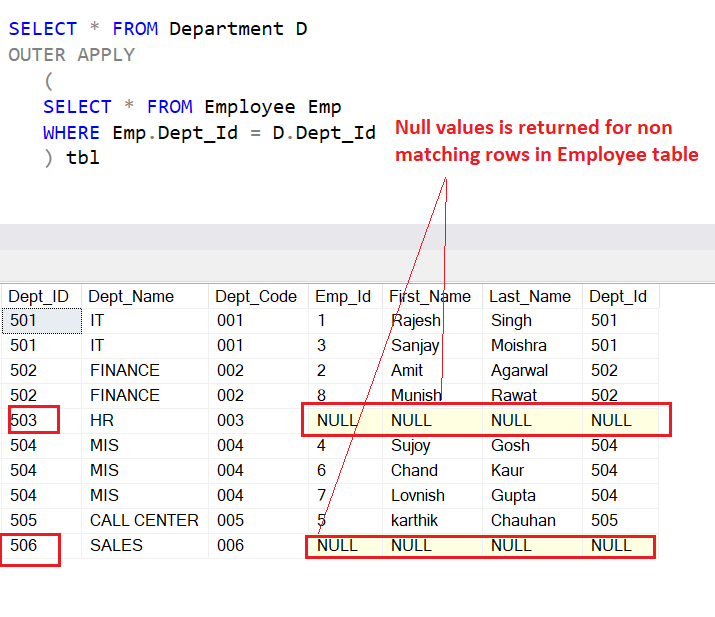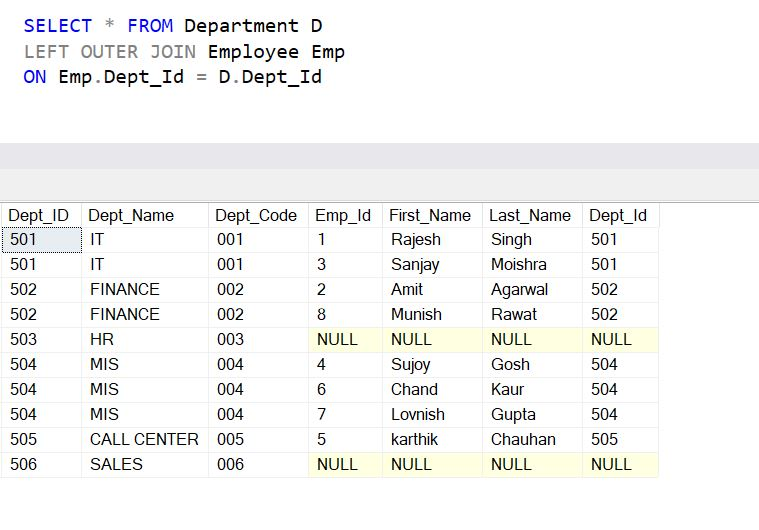Home » SQL Server Cross Apply and Outer Apply

# SQL Server Cross Apply and Outer Apply### SQL Server Cross Apply and Outer Apply

SQL Server APPLY operator is like a SQL JOINS, which allows joining two table expressions. But the difference between APPLY operator and JOINS is, the ability of APPLY operator to work with table- valued expression.

APPLY operator can deal with table-valued function, It allows us to invoke a table-valued function for each row returned by an outer table expression of a query. Means if you have a table-valued expression on the right side and you want this table-valued expression to be evaluated for each row from the left table expression. Then this can be achieved by APPLY Operator.

SQL Server provides two forms of Apply Operator that is CROSS APPLY and OUTER APPLY.

The CROSS APPLY operator returns only those rows from the left table expression which are matched with the right table expression. CROSS APPLY work as a row by row INNER JOIN.

The OUTER APPLY operator returns all the rows from the left table expression irrespective of its match with the right table expression. It contains NULL values in columns of the right table expression for those rows for which there are no corresponding matches in the right table expression. OUTER APPLY work as LEFT OUTER JOIN.

Lets look at an example of CROSS APPLY and OUTER APPLY in SQL.

First we prepare a table Department and Employee then insert some dummy records into these tables.

```CREATE TABLE [Department](
[Dept_ID] [int] NOT NULL PRIMARY KEY,
[Dept_Name] VARCHAR(100) NOT NULL,
[Dept_Code] VARCHAR(10)
)

INSERT INTO Department (Dept_Id, Dept_Name, Dept_Code)
VALUES (501, 'IT', '001'),
(502, 'FINANCE', '002'),
(503, 'HR', '003'),
(504, 'MIS', '004'),
(505, 'CALL CENTER', '005'),
(506, 'SALES', '006')

CREATE TABLE [Employee](
[Emp_Id] [int] NOT NULL PRIMARY KEY,
[First_Name] VARCHAR(250) NOT NULL,
[Last_Name] VARCHAR(250) NOT NULL,
[Dept_Id] [int] NOT NULL REFERENCES [Department](Dept_Id),
)

INSERT [Employee] ([Emp_Id], [First_Name], [Last_Name], [Dept_Id])
VALUES
(1, 'Rajesh', 'Singh', 501),
(2, 'Amit', 'Agarwal', 502),
(3, 'Sanjay', 'Moishra', 501),
(4, 'Sujoy', 'Gosh', 504),
(5, 'karthik', 'Chauhan', 505),
(6, 'Chand', 'Kaur', 504),
(7, 'Lovnish', 'Gupta', 504),
(8, 'Munish', 'Rawat', 502)```

#### CROSS APPLY

Following statement uses CROSS APPLY operator that fetches data from the Department table and uses a CROSS APPLY to evaluate the Employee table for each records of the Department table.

```SELECT * FROM Department D
CROSS APPLY
(
SELECT * FROM Employee Emp
WHERE Emp.Dept_Id = D.Dept_Id
) tbl```

As you can see the result set in below screenshot, CROSS APPLY operator returns only those rows from the left table expression which are matched with the right table expression in its final result set.

For Dept_ID 503 and 506 in Department table there are no matching rows in Employee table that’s why details for these Dept_Id are not returned in result set.

The result produced by CROSS APPLY operator is same like a INNER JOIN.Lets apply INNER JOIN on same tables to see the result set.

Following statement uses INNER JOIN, which returns the records which are matched in table Department and Employee.

```SELECT * FROM Department D
INNER JOIN Employee Emp
ON Emp.Dept_Id = D.Dept_Id```

You can see the result produced by INNER JOIN that is same as CROSS APPLY Operator.So far it is fine, and we have seen CROSS APPLY acts like a SQL INNER JOIN, Lets see the ability of CROSS APPLY operator which is real purpose, or need of using CROSS APPLY operator that is dealing with table-valued function.

#### Using CROSS APPLY with Table- Valued Function

Lets say we have a table- valued function which takes Dept_Id as an input and returns employee’s details based on that dept_id.

Lets create a table- valued function – fn_GetEmployeesDetailsByDept

```CREATE FUNCTION dbo.fn_GetEmployeesDetailsByDept(@Dept_Id AS INT)
RETURNS TABLE
AS
RETURN
(
SELECT * FROM Employee
WHERE Dept_id= @Dept_Id
)
GO```

Following statement fetches records from Department table and uses a CROSS APPLY to join with the table- valued function.

It passes the Dept_Id for each row from the outer table expression that is Department table, and evaluates  the function for each row.

```SELECT * FROM Department D
CROSS APPLY dbo.fn_GetEmployeesDetailsByDept(D.Dept_Id)```

#### OUTER APPLY

Following statement fetches data from Department table and uses an OUTER APPLY to evaluate the Employee table for each record of the Department table, and non matching rows in the Employee table, contain NULL values.

You can see for Dept_Id 503 and 506 in Department table there are no matching rows in Employee table so null values are returned for employee tables in the result set.

The result produced by the Outer Apply is same like a SQL LEFT OUTER JOIN

```SELECT * FROM Department D
OUTER APPLY
(
SELECT * FROM Employee Emp
WHERE Emp.Dept_Id = D.Dept_Id
) tbl```Lets see the result produced by the Left outer join on same tables.

```SELECT * FROM Department D
LEFT OUTER JOIN Employee Emp
ON Emp.Dept_Id = D.Dept_Id```You can see result produced by left outer join is same as Outer Apply.

This is the similarity between Left outer join and Outer Apply, Lets see the actual use of Outer Apply.

#### Using OUTER APPLY With Table Valued Function

Following statement fetches records from Department table and uses a OUTER APPLY to join with the table- valued function.

It passes the Dept_Id for each row from the outer table expression that is Department table, evaluates  the function for each row and  returns non-correlated data where non matching rows contains NULLs.

```SELECT * FROM Department D
OUTER APPLY dbo.fn_GetEmployeesDetailsByDept(D.Dept_Id)```As you have see that APPLY Operator is useful in case you want to invoke a table-valued function for each row returned by an outer table expression of a query